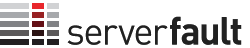## Up, accept and down votes over time

0

(click on this box to dismiss)Q&A for system and network administrators

``````select eomonth(votes.creationdate)
, sum(case when votetypeid = 1 then 1 else 0 end)  accept
, sum(case when votetypeid = 2 then 1 else 0 end)  up
, sum(case when votetypeid = 3 then 1 else 0 end)  down
, sum(case when votetypeid = 2 and posttypeid = 1 then 1 else 0 end)  [Q-up]
, sum(case when votetypeid = 3 and posttypeid = 1 then 1 else 0 end)  [Q-down]
, sum(case when votetypeid = 2 and posttypeid = 2 then 1 else 0 end)  [A-up]
, sum(case when votetypeid = 3 and posttypeid = 2 then 1 else 0 end)  [A-down]
, sum(case when votetypeid = 3 and commentcount = 0 then 1 else 0 end) [down-without-comment]Hold tight while we fetch your results